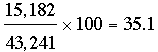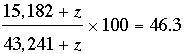SEARCH HOMEMath Central Quandaries & QueriesQuestion from Chris, a parent: I am trying to figure out how much more volume is needed to reach a certain %. For example if I sell 15,182 of unit X out of 43,241 total units. Then I know that unit X is 35.1% of units sold. However, I need to get unit X to be 46.3% of total units sold. I need to figure how many more units of X I need to sell to reach this %. Of course if I sell more units of X then this increases the denominator. Can you please help? Thank you for your time.Hi Chris,

To arrive at 35.1% as the percentage of unit X sold you performed the calculationThe question you ask is an ideal place to use a little algebra. Suppose that you sell z additional units of unit X and this results in 46.3% of the total units sold being unit X. The total sold is then 43,241 + z and the number sold of unit X is 15,182 + z. Using the same calculation as above you would haveSolve for z.

If you need additional help write back,
PennyMath Central is supported by the University of Regina and The Pacific Institute for the Mathematical Sciences.